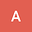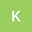Dynamical perturbation theory for eigenvalue problems
•••Maseim Kenmoe
Max Planck Institute for Mathematics in the Sciences, Leipzig, Germany, Mesoscopic and Multilayer Structures Laboratory, Department of Physics, University of Dschang, Cameroon
Author Profile## Abstract

Many problems in physics, chemistry and other fields are perturbative in nature, i.e. differ only slightly from related problems with known solutions. Prominent among these is the eigenvalue perturbation problem, wherein one seeks the eigenvectors and eigenvalues of a matrix with small off-diagonal elements. Here we introduce a novel iterative algorithm to compute these eigenpairs based on a reformulation of the eigenvalue problem as an algebraic equation in complex projective space. We show from explicit and numerical examples that our algorithm outperforms the usual Rayleigh-Schrödinger expansion on three counts. First, since it is not defined as a power series, its domain of convergence is not a priori confined to a disk in the complex plane and typically extends beyond the standard perturbative radius of convergence. Second, when both methods converge, ours does so at a faster rate, i.e. fewer orders are required to reach a given precision. Third, the (time- and space-) algorithmic complexity of each iteration does not increase with the order of the approximation, allowing for higher precision computations. Because this complexity is merely that of matrix multiplication, our dynamical scheme also scales better with the size of the matrix than general-purpose eigenvalue routines such as the shifted QR or divide-and-conquer algorithms. Whether they are dense, sparse, symmetric or unsymmetric, we confirm that dynamical diagonalization quickly outpaces LAPACK drivers as the size of matrices grows; for the computation of just the dominant eigenvector, our method converges order of magnitudes faster than the Arnoldi algorithm implemented in ARPACK.